What does it do?

Creates a cell reference based on the row and column numbers

Formula breakdown:

What it means:

=ADDRESS(row number, column number, [absolute or relative], [reference style], [name of the worksheet])

Did you know that you can dynamically create cell references in Excel? Yes you can with the ADDRESS Formula! This is one of the Lookup Formulas in Excel.

The ADDRESS Formula takes in these information to create the cell reference:

• row number
• column number
• abs_num – this is reflected if your cell reference is absolute or relative. It has 4 possibilities:
• 1 – Absolute
• 2 – Absolute row, Relative column
• 3 – Relative row, Absolute column
• 4 – Relative
• a1 – this determines if it’s R1C1 or A1 style. For our examples we will not use this, and it will default to A1 Style
• 0 – R1C1 Style
• 1 – A1 Style
• sheet_text – this will add the sheet name to your cell reference if populated

I explain how you can do this below:STEP 1: We need to enter the ADDRESS function in a blank cell: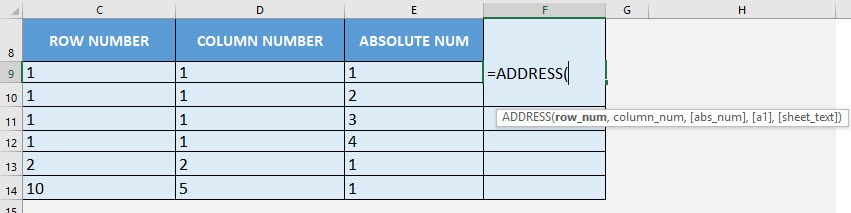## row_num

What is the row number?

Select the cell containing the row number: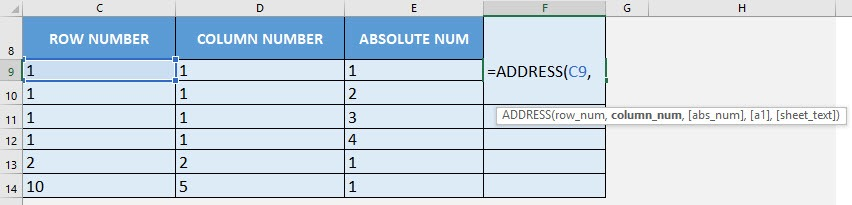## column_num

What is the column number?

Select the cell containing the column number: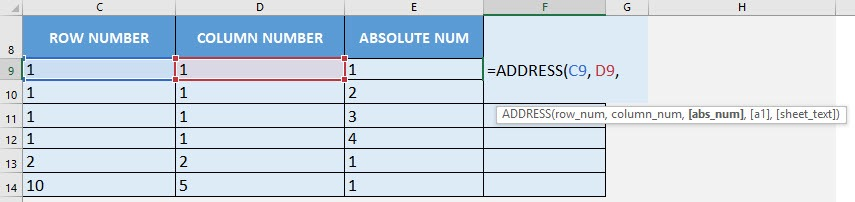## abs_num

Would it be an absolute or relative cell reference?

Select the cell containing the abs_num input. There are 4 modes, so we have included in all of the examples so that you can see it in action.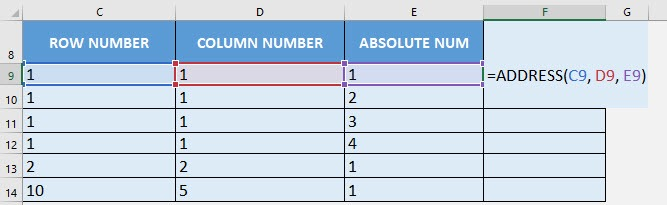Apply the same formula to the rest of the cells by dragging the lower right corner downwards.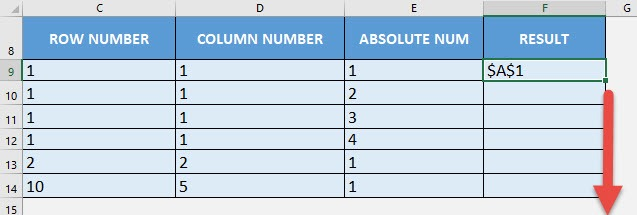You have all of your cell references generated now! Notice the differences in the 4 modes as well represented by the \$A\$1 cell references: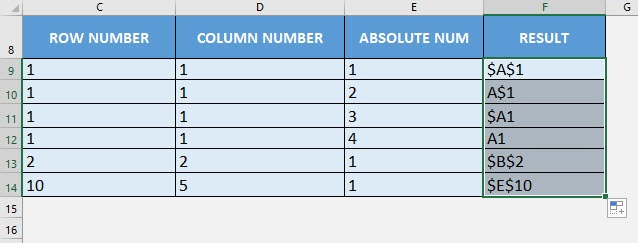How to Use the ADDRESS Formula in Excel